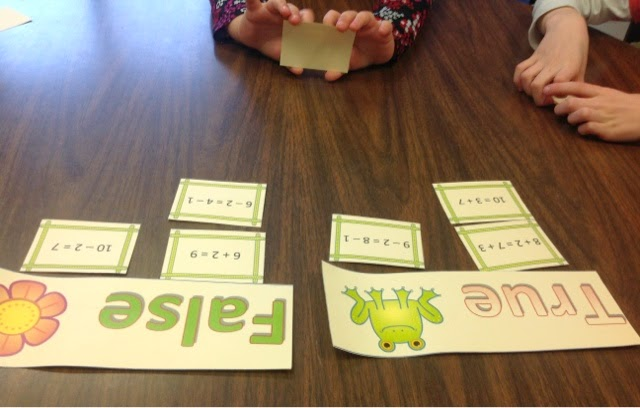## Saturday, April 12, 2014

### Working on Equality

If you have spent any time teaching math to young kids or have spent any time in a middle school setting, you are probably familiar with the idea that kids really do not know what the equals sign means.  Many students see it as operational meaning here comes the answer.  It is so important for kids to be exposed to ideas of the equals sign meaning equality from a young age.  I have written before about how I have worked on equality here and here if you are looking for ideas to get started.  Today, I want to share with you a recent experience with first graders.

We were working on this Common Core Standard
CCSS.MATH.CONTENT.1.OA.D.7
Understand the meaning of the equal sign, and determine if equations involving addition and subtraction are true or false. For example, which of the following equations are true and which are false? 6 = 6, 7 = 8 - 1, 5 + 2 = 2 + 5, 4 + 1 = 5 + 2.Understanding this standard directly supports this one and the development of good solid strategies for addition and subtraction facts.

CCSS.MATH.CONTENT.1.OA.C.6
Add and subtract within 20, demonstrating fluency for addition and subtraction within 10. Use strategies such as counting on; making ten (e.g., 8 + 6 = 8 + 2 + 4 = 10 + 4 = 14); decomposing a number leading to a ten (e.g., 13 - 4 = 13 - 3 - 1 = 10 - 1 = 9); using the relationship between addition and subtraction (e.g., knowing that 8 + 4 = 12, one knows 12 - 8 = 4); and creating equivalent but easier or known sums (e.g., adding 6 + 7 by creating the known equivalent 6 + 6 + 1 = 12 + 1 = 13).

I get out my trusty math balance for this activity and start by having kids make some equations that are true.  We then jump into making other equations, recording them on the board and deciding if they are true or false.  I then ask kids to come up with a true equation or come up with a false equation and we test their idea together.  As you can imagine, kids love making up equations that are false.Here is the start of our equation list.  Students write them on the white board and record T for true or F for false.Look at all the different types of equations kids have come up with!We use the math balance to check out ideas and verify if equations are true or false.
After we have done this whole group activity, it is time for partner work.  I used my Frog and Flower Equality cards to differentiate this partner work for kids.  These cards are offered at 4 different levels so I can make it easier or harder for kids depending on their own levels.  I can also offer kids who are ready more of a challenge or a second set to do when they are ready for it.Students read the equations and sort them into true or false!

How do you work on equality with your students?